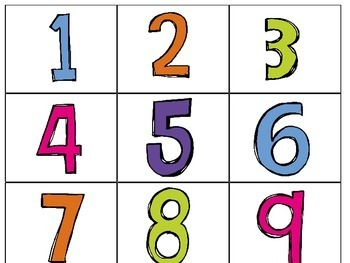# Ten Frames Center Activity

Rated 5 out of 5, based on 5 reviews
5 Ratings;
PreK - 1st
Subjects
Resource Type
Standards
Formats Included
• PDF
Pages
57 pages
Report this resource to TPT

### Description

Need a fun way to teach number quantities? Have your students practice number words as well as one to one correspondence with this matching game. Students match the number to the word, and the quantity.

<<<<<<<<<<<<<<<<<<<<<<<<<<<<<<<<<<<<<<<<<<<<<<<<<<<<<<<<<<<<<<<<<<<<<

<<<<<<<<<<<<<<<<<<<<<<<<<<<<<<<<<<<<<<<<<<<<<<<<<<<<<<<<<<<<<<<<<<<<<

Subitizing Math Centers:

Ten Frame and Tally Mark {April}

Other products perfect for math centers:

<<<<<<<<<<<<<<<<<<<<<<<<<<<<<<<<<<<<<<<<<<<<<<<<<<<<<<<<<<<<<<<<<<<<<

Don't forget that leaving feedback earns you points toward FREE TPT purchases. I love that feedback!

Also, follow me and be notified when new products are uploaded. New products are always 50% off for the first 24 hours they are posted. It pays to follow me!

Thank you,

Teacherof20

Total Pages
57 pages
N/A
Teaching Duration
N/A
Report this resource to TPT
Reported resources will be reviewed by our team. Report this resource to let us know if this resource violates TPT’s content guidelines.

### Standards

to see state-specific standards (only available in the US).
Compose and decompose numbers from 11 to 19 into ten ones and some further ones, e.g., by using objects or drawings, and record each composition or decomposition by a drawing or equation (e.g., 18 = 10 + 8); understand that these numbers are composed of ten ones and one, two, three, four, five, six, seven, eight, or nine ones.
10 can be thought of as a bundle of ten ones - called a “ten.”
The numbers from 11 to 19 are composed of a ten and one, two, three, four, five, six, seven, eight, or nine ones.